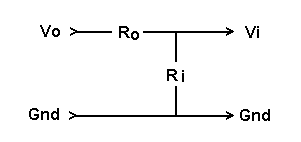Daqarta
Data AcQuisition And Real-Time Analysis
Scope - Spectrum - Spectrogram - Signal Generator
Software for WindowsThe following is from the Daqarta Help system:

# Full-Scale Range - Output Voltage Method

Controls: Calibration Menu >> Full-Scale Range

## Introduction:

This approach to Full-Scale Range calibration involves measuring the Generator output with an external voltmeter and setting the Output Range, then applying the now-calibrated signal to the input to get the Input Range.

Note: You must have previously run Auto-Calibrate for the output and input lines whose range you want to set; otherwise, their controls will be disabled in the Full-Scale Range dialog.

In addition, you should also perform input and output impedance measurements on your card, since these values will be used to correct the calibration.

## Finding Output Range:

To measure the output voltage accurately you need a separate voltmeter that has a sensitive AC Volts range, ideally one that can read 3 decimal places (1.999), or at least 2 places (19.99) AC volts full-scale.

Connect the meter to the output. If you are clipping the meter leads to the end of a male-male cable plugged into the output, the red lead goes to the tip (Left channel) and the black lead goes to the shank (common or ground). Don't try to clip onto the little ring between the tip and shank (Right channel), since it's hard to avoid shorting things together. This procedure only uses the Left channel, and Daqarta assumes the Left and Right calibrations are identical.

Set the Generator to produce a sine wave at maximum volume and 100% Level. The frequency should be in the range of 50 to 1000 hertz for best accuracy on most meters. The reading from the meter will be an RMS value, which you must convert to amplitude by multiplying by 1.4142 (the square root of 2). Enter that value for Wave Out in the Range dialog.

Alternatively, you can skip the RMS-to-amplitude conversion and compare the Daqarta Voltmeter RMS reading with the external meter RMS reading. You might want to do this if you are tweaking a prior calibration, or especially if you want to run the output at a softer volume because it distorts at the maximum setting (see below). Note that you can use lower volumes only because you have done a relative calibration with Auto-Calibrate.

Just open the Daqarta Voltmeter (ALT+V) and set the channel to Left Out and the mode to RMS. The true Wave Out range will then be the current Wave Out range (default 1.000) times the external meter reading divided by the Daqarta Voltmeter reading, both in RMS volts.

True_Range = Current_Range * V_Ext / V_Daqarta

## Finding Input Range:

Now Daqarta has become a calibrated signal generator which you can use as a reference signal source to calibrate the inputs. Use a "loopback" connection (as in Auto-Calibrate) to connect the outputs to the inputs. In the Input dialog make sure both Left and Right channels are active. Select Line In and set the Line In level to 0.

Adjust the output volume until you get a large (nearly full-scale) input, without over-driving it into distortion. One easy way to do this is to monitor the spectrum instead of the waveform; when you drive the input too hard, you will see a sudden onset or rise of spectral peaks at harmonic frequencies. This is much more sensitive than judging sine-wave distortion by viewing the waveform.

It is unfortunately all too common for sound card inputs to distort well before the input signal reaches full-scale... sometimes as little as half-scale.

Now set the Daqarta Voltmeter to Left In and read the RMS voltage. Since Daqarta is assuming whatever Input Range value is currently set (default 1.000 volt), this "apparent" voltage is unlikely to be the true input voltage... yet.

Now read the signal level that produces that input voltage by toggling the Daqarta Voltmeter from Left In to Left Out. To find the true full-scale input range, multiply the current Range by the output voltage divided by the apparent input voltage.

Test_RangeIn = Current_RangeIn * Vout / Vin

For example, suppose the true output is 250 mV where the apparent input is 0.600 volt with the default full-scale range of 1.000 volt. Then the correct full-scale value will be:

1.000 * 0.250 / 0.600 = 0.4167

However, the above calculation does not consider the sound card output and input impedances. The situation is a voltage divider that reduces the true input voltage Vi at the sound card's ADC, relative to the true no-load sound card output Vo:The value calculated above assumed output impedance Ro was zero. The true value has been reduced by the divider according to:

Ri / (Ro + Ri)

So to find the correct value, which we would have gotten if the assumptions were true, we multiply the computed value by the reciprocal of the above:

True_RangeIn = Test_RangeIn * (Ro + Ri) / Ri

Enter the True_RangeIn value as the Stereo Range value for that Input line.

Double that value and enter it for Mono. That's the value Daqarta will use when only the Left or Right Input channel is selected; it will be OK for most cards, since they sum the two inputs together in that case. But note that if you have separate Left and Right Input level controls, each will be applied to its respective channel, even though one channel is apparently not selected.

This makes Mono calibration very tricky to use. It's much better to always use both channels, even if you have no signal applied to one of them.GO: